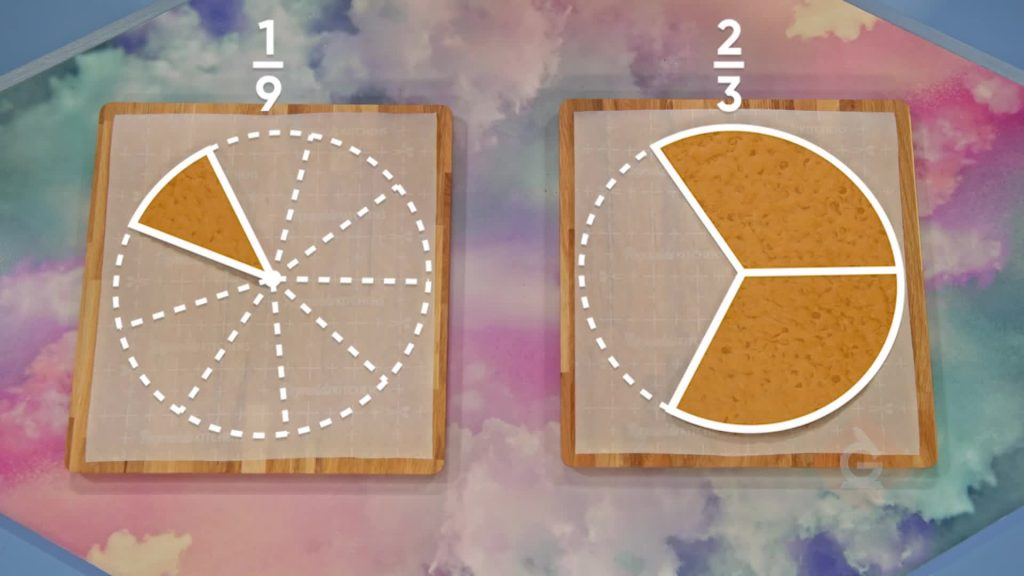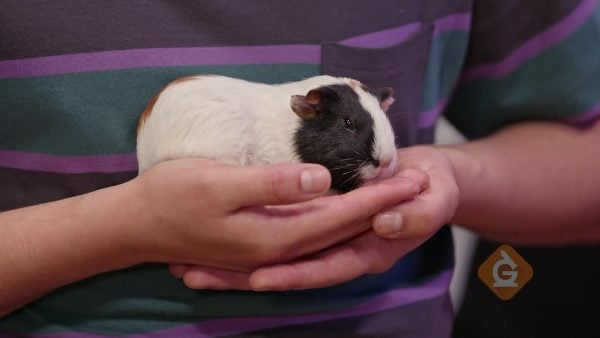Add & Subtract Fractions (Unlike Denominators) Math Video | Grades 3-5
1%
It was processed successfully!WHAT IS ADDING & SUBTRACTING FRACTIONS WITH UNLIKE DENOMINATORS?

Two fractions have unlike denominators if the numbers in the denominators are not the same. You can use multiplication and division to rewrite fractions to have a common denominator.

To better understand adding and subtracting fractions with unlike denominators…

WHAT IS ADDING & SUBTRACTING FRACTIONS WITH UNLIKE DENOMINATORS?. Two fractions have unlike denominators if the numbers in the denominators are not the same. You can use multiplication and division to rewrite fractions to have a common denominator. To better understand adding and subtracting fractions with unlike denominators…

## LET’S BREAK IT DOWN!### Algorithm for Finding a Common DenominatorLet's say you want to add [ggfrac]1/3[/ggfrac]+ [ggfrac]2/5[/ggfrac]. You know that you need to find a common denominator. Starting with [ggfrac]1/3[/ggfrac], list equivalent fractions: [ggfrac]1/3[/ggfrac] × [ggfrac]2/2[/ggfrac] = [ggfrac]2/6[/ggfrac], [ggfrac]1/3[/ggfrac] × [ggfrac]3/3[/ggfrac]= [ggfrac]3/9[/ggfrac], [ggfrac]1/3[/ggfrac] × [ggfrac]4/4[/ggfrac] = [ggfrac]4/12[/ggfrac], [ggfrac]1/3[/ggfrac] × [ggfrac]5/5[/ggfrac] = [ggfrac]5/15[/ggfrac], [ggfrac]1/3[/ggfrac] × [ggfrac]6/6[/ggfrac] = [ggfrac]6/18[/ggfrac]. None of those fractions have a denominator of 5, so you need to find equivalent fractions for [ggfrac]2/5[/ggfrac] as well: [ggfrac]2/5[/ggfrac] × [ggfrac]2/2[/ggfrac]= [ggfrac]4/10[/ggfrac], [ggfrac]2/5[/ggfrac] × [ggfrac]3/3[/ggfrac]= [ggfrac]6/15[/ggfrac], [ggfrac]2/5[/ggfrac] × [ggfrac]4/4[/ggfrac]= [ggfrac]8/20[/ggfrac]. Both [ggfrac]1/3[/ggfrac] and [ggfrac]2/5[/ggfrac] have an equivalent fraction with a denominator of 15. Rewrite the original addition as [ggfrac]5/15[/ggfrac]+ [ggfrac]6/15[/ggfrac]= [ggfrac]11/15[/ggfrac].

There is a faster way to find a common denominator. If you multiply the numerator and the denominator of each fraction by the denominator of the other fraction, you always get fractions with a common denominator. Here's how this works: Starting with [ggfrac]1/3[/ggfrac], you see that the other fraction, [ggfrac]2/5[/ggfrac], has a denominator of 5 so you multiply the numerator and denominator by 5: [ggfrac]1/3[/ggfrac] × [ggfrac]5/5[/ggfrac] = [ggfrac]5/15[/ggfrac]. Next, you see that [ggfrac]1/3[/ggfrac] has a denominator of 3, so you multiply the numerator and denominator of the other fraction by 3: [ggfrac]2/5[/ggfrac] × [ggfrac]3/3[/ggfrac]= [ggfrac]6/15[/ggfrac]. Rewrite the original addition as [ggfrac]5/15[/ggfrac]+ [ggfrac]6/15[/ggfrac]= [ggfrac]11/15[/ggfrac]. Try this one yourself. Add [ggfrac]1/6[/ggfrac] + [ggfrac]3/4[/ggfrac] by finding a common denominator using the faster way.

Algorithm for Finding a Common Denominator Let's say you want to add [ggfrac]1/3[/ggfrac]+ [ggfrac]2/5[/ggfrac]. You know that you need to find a common denominator. Starting with [ggfrac]1/3[/ggfrac], list equivalent fractions: [ggfrac]1/3[/ggfrac] × [ggfrac]2/2[/ggfrac] = [ggfrac]2/6[/ggfrac], [ggfrac]1/3[/ggfrac] × [ggfrac]3/3[/ggfrac]= [ggfrac]3/9[/ggfrac], [ggfrac]1/3[/ggfrac] × [ggfrac]4/4[/ggfrac] = [ggfrac]4/12[/ggfrac], [ggfrac]1/3[/ggfrac] × [ggfrac]5/5[/ggfrac] = [ggfrac]5/15[/ggfrac], [ggfrac]1/3[/ggfrac] × [ggfrac]6/6[/ggfrac] = [ggfrac]6/18[/ggfrac]. None of those fractions have a denominator of 5, so you need to find equivalent fractions for [ggfrac]2/5[/ggfrac] as well: [ggfrac]2/5[/ggfrac] × [ggfrac]2/2[/ggfrac]= [ggfrac]4/10[/ggfrac], [ggfrac]2/5[/ggfrac] × [ggfrac]3/3[/ggfrac]= [ggfrac]6/15[/ggfrac], [ggfrac]2/5[/ggfrac] × [ggfrac]4/4[/ggfrac]= [ggfrac]8/20[/ggfrac]. Both [ggfrac]1/3[/ggfrac] and [ggfrac]2/5[/ggfrac] have an equivalent fraction with a denominator of 15. Rewrite the original addition as [ggfrac]5/15[/ggfrac]+ [ggfrac]6/15[/ggfrac]= [ggfrac]11/15[/ggfrac]. There is a faster way to find a common denominator. If you multiply the numerator and the denominator of each fraction by the denominator of the other fraction, you always get fractions with a common denominator. Here's how this works: Starting with [ggfrac]1/3[/ggfrac], you see that the other fraction, [ggfrac]2/5[/ggfrac], has a denominator of 5 so you multiply the numerator and denominator by 5: [ggfrac]1/3[/ggfrac] × [ggfrac]5/5[/ggfrac] = [ggfrac]5/15[/ggfrac]. Next, you see that [ggfrac]1/3[/ggfrac] has a denominator of 3, so you multiply the numerator and denominator of the other fraction by 3: [ggfrac]2/5[/ggfrac] × [ggfrac]3/3[/ggfrac]= [ggfrac]6/15[/ggfrac]. Rewrite the original addition as [ggfrac]5/15[/ggfrac]+ [ggfrac]6/15[/ggfrac]= [ggfrac]11/15[/ggfrac]. Try this one yourself. Add [ggfrac]1/6[/ggfrac] + [ggfrac]3/4[/ggfrac] by finding a common denominator using the faster way.

### Guinea Pig WeightsLet's say that you have two tiny guinea pigs. One weighs [ggfrac]4/5[/ggfrac] of a pound and the other weighs [ggfrac]2/3[/ggfrac] of a pound. You want to figure out how much more one guinea pig weighs than the other. You can find this difference using the equation [ggfrac]4/5[/ggfrac] - [ggfrac]2/3[/ggfrac]= ?. First, find equivalent fractions that have a common denominator. The second fraction has a denominator of 3, so multiply the numerator and denominator of the first fraction by 3. [ggfrac]4/5[/ggfrac] × [ggfrac]3/3[/ggfrac] = [ggfrac]12/55[/ggfrac]. Next, multiply [ggfrac]2/3[/ggfrac] × [ggfrac]5/5[/ggfrac] since 5 is the denominator of [ggfrac]4/5[/ggfrac]. [ggfrac]2/3[/ggfrac] × [ggfrac]5/5[/ggfrac]= [ggfrac]10/15[/ggfrac]. Rewrite the original equation [ggfrac]4/5[/ggfrac] - [ggfrac]2/3[/ggfrac] as [ggfrac]12/15[/ggfrac] - [ggfrac]10/15[/ggfrac]= [ggfrac]2/15[/ggfrac]. One guinea pig weighs [ggfrac]2/15[/ggfrac] of a pound more than the other guinea pig. Try this one yourself. My bag of candy weighs [ggfrac]3/4[/ggfrac] of a pound and my friend's bag of candy weighs [ggfrac]2/3[/ggfrac] of a pound. How much more does my bag of candy weigh?

Guinea Pig Weights Let's say that you have two tiny guinea pigs. One weighs [ggfrac]4/5[/ggfrac] of a pound and the other weighs [ggfrac]2/3[/ggfrac] of a pound. You want to figure out how much more one guinea pig weighs than the other. You can find this difference using the equation [ggfrac]4/5[/ggfrac] - [ggfrac]2/3[/ggfrac]= ?. First, find equivalent fractions that have a common denominator. The second fraction has a denominator of 3, so multiply the numerator and denominator of the first fraction by 3. [ggfrac]4/5[/ggfrac] × [ggfrac]3/3[/ggfrac] = [ggfrac]12/55[/ggfrac]. Next, multiply [ggfrac]2/3[/ggfrac] × [ggfrac]5/5[/ggfrac] since 5 is the denominator of [ggfrac]4/5[/ggfrac]. [ggfrac]2/3[/ggfrac] × [ggfrac]5/5[/ggfrac]= [ggfrac]10/15[/ggfrac]. Rewrite the original equation [ggfrac]4/5[/ggfrac] - [ggfrac]2/3[/ggfrac] as [ggfrac]12/15[/ggfrac] - [ggfrac]10/15[/ggfrac]= [ggfrac]2/15[/ggfrac]. One guinea pig weighs [ggfrac]2/15[/ggfrac] of a pound more than the other guinea pig. Try this one yourself. My bag of candy weighs [ggfrac]3/4[/ggfrac] of a pound and my friend's bag of candy weighs [ggfrac]2/3[/ggfrac] of a pound. How much more does my bag of candy weigh?Let's look at an example of subtracting mixed numbers. Let's say Marcos had a long jump of 5[ggfrac]1/6[/ggfrac] feet and April jumped 4[ggfrac]2/3[/ggfrac] feet. We can figure out how much farther Marco jumped by solving 5[ggfrac]1/6[/ggfrac]- 4[ggfrac]2/3[/ggfrac]= ?. Start by finding [ggfrac]1/6[/ggfrac] - [ggfrac]2/3[/ggfrac]. The denominators are unlike, so we need to find equivalent fractions with a common denominator: 2 × 3 = 6, so multiply the numerator and denominator of [ggfrac]2/3[/ggfrac] by 2: [ggfrac]2/3[/ggfrac] × [ggfrac]2/2[/ggfrac]= [ggfrac]4/6[/ggfrac]. The fraction subtraction is now [ggfrac]1/6[/ggfrac] - [ggfrac]4/6[/ggfrac]. 4 is greater than 1, so we need to regroup from 5 to make a fraction greater than [ggfrac]4/6[/ggfrac]. Regroup [ggfrac]1/1[/ggfrac] into [ggfrac]6/6[/ggfrac]. 5 = 4[ggfrac]6/6[/ggfrac] . Add the new sixths to [ggfrac]1/6[/ggfrac]: [ggfrac]6/6[/ggfrac]+[ggfrac]1/6[/ggfrac] =[ggfrac]7/6[/ggfrac]. Now we can subtract the fractions: [ggfrac]7/6[/ggfrac] - [ggfrac]4/6[/ggfrac]= [ggfrac]3/6[/ggfrac]. Finally, subtract the remaining whole number parts of the mixed numbers: 4 – 4 = 0. Marcos jumped [ggfrac]3/6[/ggfrac] of a foot farther than April. Try this one yourself. I hiked 6[ggfrac]1/8[/ggfrac] miles on Saturday and another 4[ggfrac]2/3[/ggfrac] miles on Sunday. How many total miles did I hike this weekend?

## ADD & SUBTRACT FRACTIONS (UNLIKE DENOMINATORS) VOCABULARY

Numerator
In a fraction, the number of equal parts being considered.
Denominator
In a fraction, the number of equal-size parts that a whole has been split into.
Equivalent Fractions
Fractions with different numerators and denominators that name the same number.
Like Denominator
The same denominator.
Unlike Denominator
Different denominators.
Benchmark Number
Numbers such as 0, [ggfrac]1/2[/ggfrac], and 1 that can be used to estimate an answer or evaluate the reasonableness of an answer.
A number that is written using a whole number and a fraction.

## ADD & SUBTRACT FRACTIONS (UNLIKE DENOMINATORS) DISCUSSION QUESTIONS

The denominator.

### What is the meaning of the numerator when adding or subtracting fractions?

The number of equal-sized pieces being added or subtracted.

### How can we find equivalent fractions with a common denominator for two fractions with unlike denominators?

For each fraction, multiply the numerator and denominator by a number so that the denominators of both fractions are equal. One possible multiplier is the denominator of the other fraction.

### Which of the following benchmark numbers—0, [ggfrac]1/2[/ggfrac], or 1—can you use to help estimate when adding [ggfrac]4/9[/ggfrac] to another fraction?

[ggfrac]1/2[/ggfrac].

### Rafay says that 5 - 3[ggfrac]1/3[/ggfrac] = 2[ggfrac]1/3[/ggfrac]. Is Rafay correct? If not, what did Rafay do wrong?

No; Rafay subtracted the whole number parts but didn't subtract [ggfrac]1/3[/ggfrac] from 5. He should have regrouped to get 4[ggfrac]3/3[/ggfrac] - 3[ggfrac]1/3[/ggfrac] = 1[ggfrac]2/3[/ggfrac].
X

## Success

We’ve sent you an email with instructions how to reset your password.
Ok